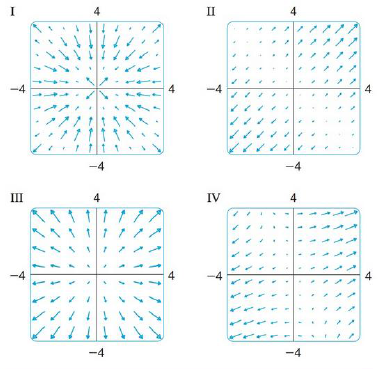Chapter 16.1, Problem 30E

Chapter
Section
Textbook Problem

Match the functions f with the plots of their gradient vector fields labeled I-IV. Give reasons for your choices.30. f(x, y) = x(x + y)To determine

To match: The function f(x,y)=x(x+y) with the plots labeled as I-IV.

Explanation

Given data:

The function is f(x,y)=x(x+y).

Formula used:

The gradient vector field of two dimensional vector is given by,

f(x,y)=fxi+fyj

Calculation:

Find the gradient vector field of f(x,y)=x(x+y), by the use of above mentioned formula as,

f(x,y)=x(x(x+y))i+y(x(x+y))j=x(x2+xy)i+y(x2+xy)j (1)

Differentiate the term x2+xy with respect to x as,

x(x2+xy)=x(x2)+x(xy)=2x+y

Differentiate the term x2+xy with respect to y

Still sussing out bartleby?

Check out a sample textbook solution.

See a sample solution

The Solution to Your Study Problems

Bartleby provides explanations to thousands of textbook problems written by our experts, many with advanced degrees!

Get Started

Place the following set of n = 20 scores in a frequency distribution table.

Essentials of Statistics for The Behavioral Sciences (MindTap Course List)

Proof Prove that sinh1t=ln(t+t2+1)

Calculus: Early Transcendental Functions (MindTap Course List)

The directrix of the conic given by r=62+10sin is: a) x=53 b) x=35 c) y=53 d) y=35

Study Guide for Stewart's Single Variable Calculus: Early Transcendentals, 8th